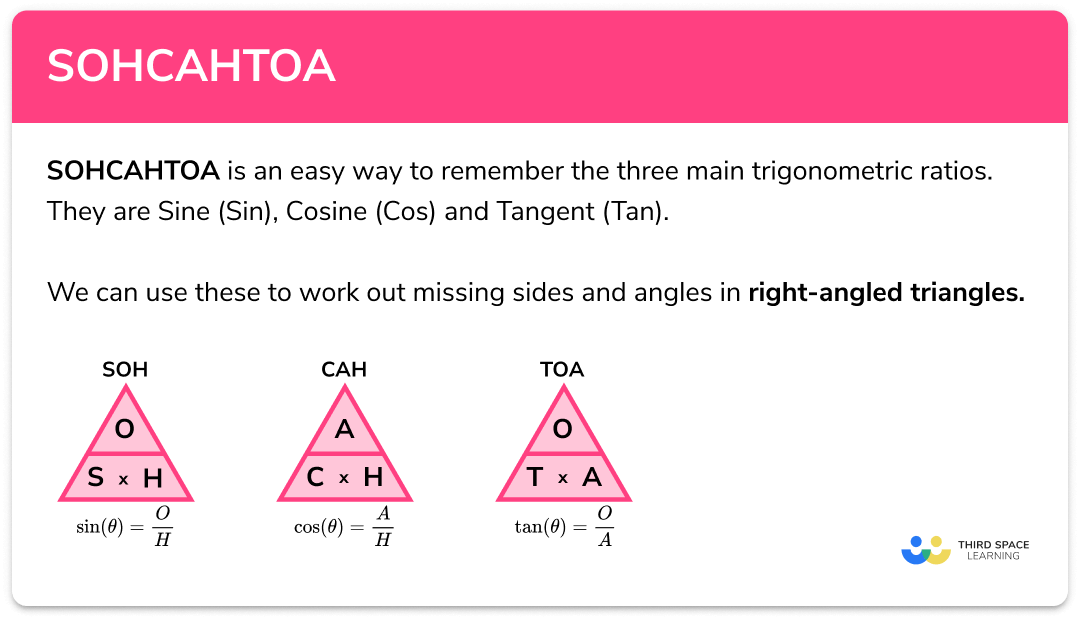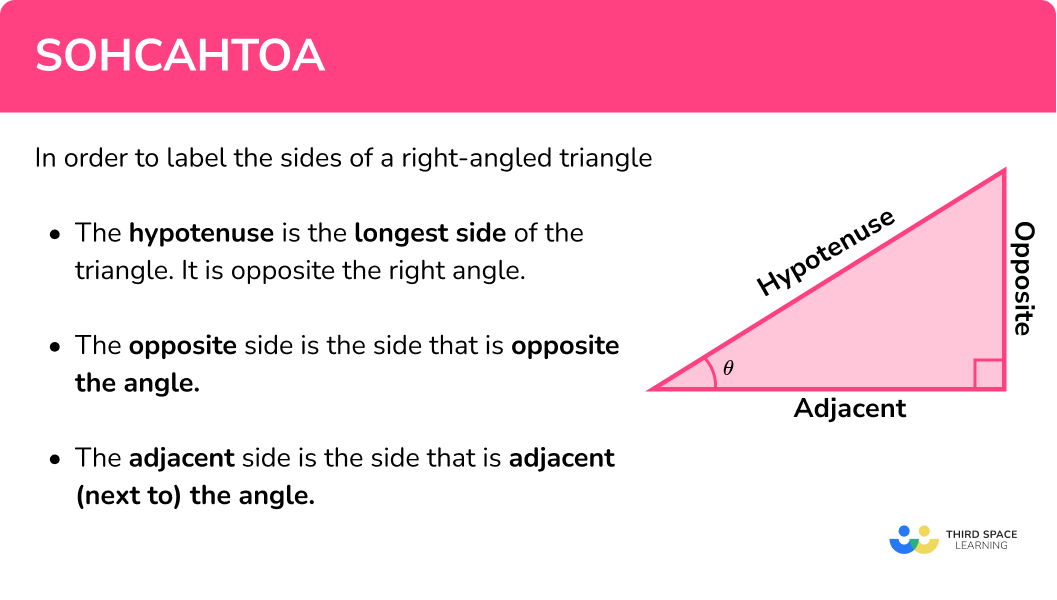# SOHCAHTOA

Here we will learn how to use SOHCAHTOA and trigonometry to find unknown sides and angles in right angled triangles. You’ll learn how to label the sides of right-angled triangles, what sin, cos and tan are, what their inverses are (sin-1, cos-1, tan-1) and how we can use them.

Look out for the trigonometry practice problems, worksheets and exam questions at the end.

## What is SOHCAHTOA?

SOHCAHTOA is a mnemonic that gives us an easy way to remember the three main trigonometric ratios. They are sine (sin), cosine (cos) and tangent (tan).

We can use these to work out missing sides and angles in right-angled triangles

### What is SOHCAHTOA?## What are the sohcahtoa rules?

• SOH: Sine = Opposite / Hypotenuse
$\sin ( \theta ) = \frac{Opposite}{Hypotenuse}$

• CAH: Cosine = Adjacent / Hypotenuse
$\cos ( \theta ) = \frac{Adjacent}{Hypotenuse}$

• TOA: Tangent = Opposite / Adjacent
$\tan ( \theta ) = \frac{Opposite}{Adjacent}$

We can abbreviate these to the SOHCAHTOA triangles:

## How to label the sides of a right angled triangle

The hypotenuse is the longest side of the triangle. It is opposite the right angle.

The opposite side is the side that is opposite the angle.

The adjacent side is the side that is adjacent (next to) the angle.

E.g.
We have a right-angled triangle with a side of length 8 cm and a side of length x cm

Label the sides of the right-angled triangle.

1. Label the longest side of the triangle H (hypotenuse)

2Label the side opposite the angle O (opposite)

3Label the side next to the angle A (adjacent)

Top tip: once you have labeled the hypotenuse (H) and the opposite (O), the adjacent (A) must be the only side left!

### How to label the sides of a right angled triangle## How to use SOHCAHTOA to find the unknown sides of a right angled triangle

We can use trigonometry to work out the unknown sides of a right-angled triangle by using SOHCAHTOA.

1. Label the sides of the right-angled triangle that we have information about.
2. Choose the trig ratio we need.
3. Substitute the values from the triangle into the function.
4. Calculate the unknown side, rearranging if necessary.

## SOHCAHTOA – unknown side examples

### Example 1: find a missing side

Calculate the side labeled x

1. Label the sides of the right-angled triangle that we have information about. Circle the labels so not to confuse them with the side lengths.

2Choose the trig ratio we need.

The trigonometric function that involves the opposite (O) and the hypotenuse (H) is sin.

$\sin(\theta)= \frac{Opposite}{Hypotenuse}$

3Substitute the values from the triangle into the function.

$\sin(40)= \frac{x}{8}$

4Calculate the unknown side, rearranging if necessary. We can either solve the equation or use the SOHCAHTOA triangles.

x=5.1 (1 d.p)

Always check that the answer is sensible:

As 5.1 is smaller than the 8 (the hypotenuse) it is a sensible answer.

### Example 2: find a missing side

Calculate the side labeled x

The trigonometric function that involves the adjacent (A) and the hypotenuse (H) is cos.

$\cos(\theta) = \frac{Adjacent}{Hypotenuse}$

$\cos(36) = \frac{x}{15}$

x=12.1 (1.d.p)

Always check that the answer is sensible:

As 12.1 is smaller than the 15 (the hypotenuse) it is a sensible answer.

### Example 3: find a missing side

Calculate the side labeled x

The trigonometric function that involves the opposite (O) and the adjacent (A) is tan.

$\tan(\theta) = \frac{Opposite}{Adjacent}$

$\tan(36) = \frac{10}{x}$

x=13.8 (1.d.p)

Always check that the answer is sensible:

A good sketch tells us this answer looks reasonable.

### Practice SOHCAHTOA questions (finding an unknown side)

1. Calculate the length of the side labeled x . Give your answer to 1 d.p.7.8 cm18.4 cm60.4 cm16.9 cmLabel the triangle:We know H and we want to work out O so use sin.

\begin{aligned} \sin(\theta)&=\frac{O}{H}\\ \sin(23)&=\frac{x}{20}\\ 20 \times \sin(23) &= x\\ 7.81462257 &= x\\ x&=7.8\mathrm{cm} \end{aligned}

2. Calculate the length of the side labeled x . Give your answer to 2 d.p.22.42 cm37.50 cm20.18 cm11.15 cmLabel the triangle:We know A and we want to find H so use cos.

\begin{aligned} \cos(\theta)&=\frac{A}{H}\\ \cos(42)&=\frac{15}{x}\\ x \times \cos(42) &=15\\ x &= \frac{15}{\cos(42)}\\ x &=20.18449094\\ x &=20.18 \mathrm{cm} \end{aligned}

3. Calculate the length of the side labeled x . Give your answer to 2 s.f.6.0 cm4.8 cm11 cm2.8 cmLabel the triangle:We know O and we want to work out A so use tan.

\begin{aligned} \tan(\theta)&=\frac{O}{A}\\ \tan(62)&=\frac{5.3}{x}\\ x \times \tan(62) &= 5.3\\ x &=\frac{5.3}{\tan(62)}\\ x &= 2.818059988\\ x &= 2.8 \mathrm{cm} \end{aligned}

### Inverse trigonometric functions

In order to work out missing angles in right-angled triangles we need use the inverse trigonometric functions:

$\sin ^{-1} \qquad \cos ^{-1} \qquad \tan ^{-1}$

We can find these on the calculator by pressing SHIFT and then sin, cos or tan.

Step by step guide: Inverse trigonometric functions (coming soon)

## How to use SOHCAHTOA to find the unknown angles of right angled triangles

We can use trigonometry to work out the unknown angles of a right-angled triangle by using SOHCAHTOA.

1. Label the sides of the right-angled triangle that we have information about.
2. Choose the trig ratio we need.
3. Substitute the values from the triangle into the function.
4. Using inverse trig functions, work out the missing angle θ.

## SOHCAHTOA – unknown angle examples

### Example 4: find a missing angle

Calculate the angle labeled θ.

The trigonometric function that involves the opposite (O) and the hypotenuse (H) is sin.

$\sin(\theta) = \frac{Opposite}{Hypotenuse}$

$\sin(\theta) = \frac{5}{9}$

Remember we can get sin-1 on the calculator by pressing SHIFT and then sin.

Always check that the answer is sensible: We can estimate from the diagram that θ is an acute angle.

As 33.7o is less than 90o it is a sensible answer.

### Example 5: find a missing angle

Calculate the angle labeled θ.

The trigonometric function that involves the adjacent (A) and the hypotenuse (H) is cos.

$\cos(\theta) = \frac{Adjacent}{Hypotenuse}$

$\cos(\theta) = \frac{9}{20}$

Remember we can get cos-1 on the calculator by pressing SHIFT and then cos.

Always check that the answer is sensible: We can estimate from the diagram that θ is an acute angle.

As 63.7o is less than 90o it is a sensible answer.

### Example 6: find a missing angle

Calculate the angle labeled θ.

The trigonometric function that involves the opposite (O) and the adjacent (A) is tan.

$\tan(\theta) = \frac{Opposite}{Adjacent}$

$\tan(\theta) = \frac{15}{7}$

Remember we can get tan-1 on the calculator by pressing SHIFT and then tan.

Always check that the answer is sensible: We can estimate from the diagram that θ is an acute angle.

As 65o is less than 90o it is a sensible answer.

### Common misconceptions

• Make sure you calculator is in ‘degrees’
All angles at GCSE are measured in degrees. Make sure your calculator screen is showing a small ‘D’ at the top before using it for trigonometry. If it is showing an ‘R’ it will measure the angle in radians which are not needed until A Level.

• Right triangle
Right-angled triangles are sometimes called right triangles.

• When to use SOHCAHTOA
We can use SOHCAHTOA to find a missing side of a right angled triangle when we have another side and a given angle.

We can use SOHCAHTOA to find a missing angle of a right angled triangle when we have two given sides.

If we have two sides and we want to find the third we can use the Pythagorean Theorem a2+b2=c2 .

•  Other trig identities
The trigonometric identities we need to know at GCSE are sin, cos and tan however there are lots more.
Here are some you will see at A Level:

\frac{1}{\sin(\theta)} = \text{cosec}(\theta)

\frac{1}{\cos(\theta)} = \text{sec}(\theta)

\frac{1}{\tan(\theta)} = \text{cot}(\theta)

SOHCAHTOA is part of our series of lessons to support revision on trigonometry. You may find it helpful to start with the main trigonometry lesson for a summary of what to expect, or use the step by step guides below for further detail on individual topics. Other lessons in this series include:

### Practice SOHCAHTOA questions (finding an unknown angle)

1. Calculate the size of the angle labeled \theta . Give your answer to 3 s.f.43.3^{\circ}46.7^{\circ}36.0^{\circ}0.0126^{\circ}Label the triangle:We know O and H so use sin.

\begin{aligned} \sin(\theta)&=\frac{O}{H}\\ \sin(\theta)&=\frac{8}{11}\\ \theta&=\sin^{-1}(\frac{8}{11})\\ \theta &=46.65824177\\ \theta&=47.7^{\circ} \end{aligned}

2. Calculate the size of the angle labeled \theta . Give your answer to 2 s.f.64^{\circ}1.1^{\circ}26^{\circ}23^{\circ}Label the triangle:We know A and H so use cos.

\begin{aligned} \cos(\theta)&=\frac{A}{H}\\ \cos(\theta)&=\frac{5.4}{12.3}\\ \theta&=\cos^{-1}(\frac{5.4}{12.3})\\ \theta&=63.95835004\\ \theta&=64^{\circ} \end{aligned}

3. Calculate the size of the angle labeled \theta . Give your answer to 1 d.p.60.0^{\circ}0.5^{\circ}30.0^{\circ}26.6^{\circ}Label the triangle:We know O and A so use tan.

\begin{aligned} \tan(\theta)&=\frac{O}{A}\\ \tan(\theta)&=\frac{12.6}{25.2}\\ \theta &= \tan^{-1}(\frac{12.6}{25.2})\\ \theta&=26.56505118\\ \theta&= 26.6^{\circ} \end{aligned}

### SOHCAHTOA GCSE exam questions1. Find the size of angle a to 2.d.p.

(3 marks)

20-14=6cm

(1)

\cos(a) = \frac{6}{13}

(1)

\begin{aligned} a&=\cos^{-1}(\frac{6}{13})\\ a&=62.51^{\circ} \end{aligned}

(1)

2. Rosie is flying a kite. She holds the kite string 1m from the ground. The kite string is 10m long and the kite flies at an angle of 40^{\circ} . Calculate the height of the kite above the ground.(3 marks)

\begin{aligned} \sin(40)&=\frac{opp}{10}\\ 10\sin(40)&=opp \end{aligned}

(1)

opp=6.43m

(1)

total height:

6.43+1=7.43m

(1)

3. Length AB = 15cm

Angle ABE = 51^{\circ}Work out the length of DE.

(4 marks)

Length AE:

\begin{aligned} \tan(51)&=\frac{AE}{15}\\ 15\tan(51)&=AE \end{aligned}

(1)

AE = 18.52

(1)

Length DE:

\begin{aligned} \tan(32)&=\frac{18.52}{x}\\ x&=\frac{18.52}{tan(32)} \end{aligned}

(1)

x=29.64cm

(1)

## Learning checklist

You have now learned how to:

• Apply trigonometric ratios to find lengths in right-angled triangles in 2 dimensions
• Apply trigonometric ratios to find angles in right-angled triangles in 2 dimensions

## Still stuck?

Prepare your KS4 students for maths GCSEs success with Third Space Learning. Weekly online one to one GCSE maths revision lessons delivered by expert maths tutors.

Find out more about our GCSE maths tuition programme.